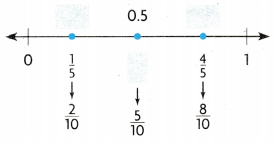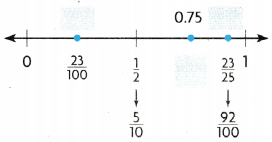Refer to our Texas Go Math Grade 4 Answer Key Pdf to score good marks in the exams. Test yourself by practicing the problems from Texas Go Math Grade 4 Module 4 Assessment Answer Key.

vocabulary

• benchmark
• numerator
• simplest form

Choose the best term from the box.

Question 1.
A ________________ is a known size or amount that you understand another size or amount.
Explanation:
A benchmark is a known size or amount that helps you understand a different size or amount. You can use 1/2 as a benchmark to help you compare fractions.

Concepts and skills

Compare. Write <, > or =.

Question 2.
$$\frac{7}{8}$$ _____________ $$\frac{7}{12}$$
Answer: $$\frac{7}{8}$$ > $$\frac{7}{12}$$
Explanation:
$$\frac{7}{8}$$ = 0.87
$$\frac{7}{12}$$ =0.58
So 0.87>0.58

Question 3.
$$\frac{10}{12}$$ _____________ $$\frac{5}{6}$$
Answer: $$\frac{10}{12}$$ = $$\frac{5}{6}$$
Explanation:
$$\frac{10}{12}$$ simplest form is $$\frac{5}{6}$$
So $$\frac{10}{12}$$ and $$\frac{5}{6}$$ are equal

Question 4.
$$\frac{1}{2}$$ _____________ $$\frac{3}{10}$$
Answer: $$\frac{1}{2}$$> $$\frac{3}{10}$$
Explanation:
$$\frac{1}{2}$$ =0.5
$$\frac{3}{10}$$ =0.3
so 0.5>0.3
Question 5.
$$\frac{1}{4}$$ _____________ $$\frac{2}{3}$$
Answer: $$\frac{1}{4}$$ < $$\frac{2}{3}$$
Explanation:
$$\frac{1}{4}$$ = 0.25
$$\frac{2}{3}$$ =0.73
So 0.25 <0.73

Question 6.
$$\frac{2}{3}$$ _____________ $$\frac{4}{7}$$
Answer: $$\frac{2}{3}$$ >$$\frac{4}{7}$$
Explanation:
$$\frac{2}{3}$$ = 0.65
$$\frac{4}{7}$$ = 0.57
So 0.65 > 0.57

Question 7.
$$\frac{5}{14}$$ _____________ $$\frac{10}{14}$$
Answer: $$\frac{5}{14}$$ <$$\frac{10}{14}$$
Explanation:
$$\frac{5}{14}$$ = 0.35
$$\frac{10}{14}$$ = 0.71
So 0.35 < 0.71

Question 8.
$$\frac{1}{4}$$ _____________ $$\frac{4}{7}$$
Answer: $$\frac{1}{4}$$ < $$\frac{4}{7}$$
Explanation:
$$\frac{1}{4}$$ = 0.25
$$\frac{4}{7}$$ = 0.57
So 0.25 < 0.57

Question 9.
$$\frac{6}{8}$$ _____________ $$\frac{1}{3}$$
Answer: $$\frac{6}{8}$$> $$\frac{1}{3}$$
Explanation:
$$\frac{6}{8}$$ = 0.75
$$\frac{1}{3}$$ = 0.33
So 0.75 > 0.33

Write the fractions in order from least to greatest.

Question 10.
$$\frac{2}{3}, \frac{3}{4}, \frac{1}{6}$$
Answer: $$\frac{1}{6}$$, $$\frac{2}{3}$$, $$\frac{3}{4}$$
Explanation:
$$\frac{2}{3}$$= 0.66
$$\frac{3}{4}$$ = 0.75
$$\frac{1}{6}$$ = 0.16
So order from least to greatest is $$\frac{1}{6}$$, $$\frac{2}{3}$$, $$\frac{3}{4}$$

Question 11.
$$\frac{7}{10}, \frac{4}{5}, \frac{1}{2}, \frac{4}{12}$$
Answer: $$\frac{4}{12}$$, $$\frac{1}{2}$$, $$\frac{7}{10}$$, $$\frac{4}{5}$$
Explanation:
$$\frac{7}{10}$$= 0.7
$$\frac{4}{5}$$ = 0.8
$$\frac{1}{2}$$ = 0.5
$$\frac{4}{12}$$ = 0.33
So order from least to greatest is $$\frac{4}{12}$$, $$\frac{1}{2}$$, $$\frac{7}{10}$$, $$\frac{4}{5}$$

Write the fraction or decimal to show their distances from zero.

Question 12.Question 13.Question 14.
Paco needs more than $$\frac{3}{8}$$ yard of twine to build a model ship. How much twine could he buy?
(A) $$\frac{3}{10}$$yard
(B) $$\frac{1}{4}$$yard
(C) $$\frac{3}{5}$$yard
(D) $$\frac{1}{8}$$yard
Answer: (C) $$\frac{3}{5}$$yard
Explanation
$$\frac{3}{8}$$ =0.38
$$\frac{3}{10}$$ =0.33
$$\frac{1}{4}$$ =0.25
$$\frac{3}{5}$$ =0.6
latex]\frac{1}{8}[/latex]=0.12
Paco needs more than 0.38 yard of twine to build a model ship. So he needs to buy $$\frac{3}{5}$$ yards which is 0.6

Question 15.
Rachel, Nancy, and Diego were in a fishing competition. Rachel’s
fish was $$\frac{7}{8}$$ foot long, Nancy’s fish was $$\frac{1}{4}$$ foot long, and Diego’s fish was $$\frac{1}{2}$$ foot long. Which shows the correct comparison of the lengths of Rachel and Diego’s fish?
(A) $$\frac{1}{4}$$ foot = $$\frac{7}{8}$$foot
(B) $$\frac{1}{2}$$ foot > $$\frac{7}{8}$$foot
(C) $$\frac{1}{2}$$ foot = $$\frac{7}{8}$$foot
(D) $$\frac{1}{2}$$ foot < $$\frac{7}{8}$$foot
Answer: (D) $$\frac{1}{2}$$ foot < $$\frac{7}{8}$$foot
Explanation:
$$\frac{7}{8}$$ = 0.88
$$\frac{1}{4}$$ = 0.25
$$\frac{1}{2}$$ =0.5
So $$\frac{1}{2}$$ foot < $$\frac{7}{8}$$foot is correcct

Question 16.
Amy needs $$\frac{6}{8}$$ gallon of fruit juice to make punch. She needs an equal amount (JI sparkling water. How much sparkling water does she need?
(A) $$\frac{2}{3}$$ gallon
(B) $$\frac{1}{2}$$ gallon
(C) $$\frac{2}{8}$$ gallon
(D) $$\frac{3}{4}$$ gallon

Question 17.
Bill has enough money to buy less than pound of cheese. How much cheese coUld Bill buy?
(A) $$\frac{4}{6}$$ pound
(B) $$\frac{5}{8}$$ pound
(C) $$\frac{1}{3}$$ pound
(D) $$\frac{3}{4}$$ pound
Answer: (D) $$\frac{3}{4}$$ pound
Explanation:
$$\frac{4}{6}$$ pound = 0.66
$$\frac{5}{8}$$ pound = 0.62
latex]\frac{1}{3}[/latex] pound = 0.33
$$\frac{3}{4}$$ pound = 0.74
So Bill can buy $$\frac{3}{4}$$ pound

Scroll to Top